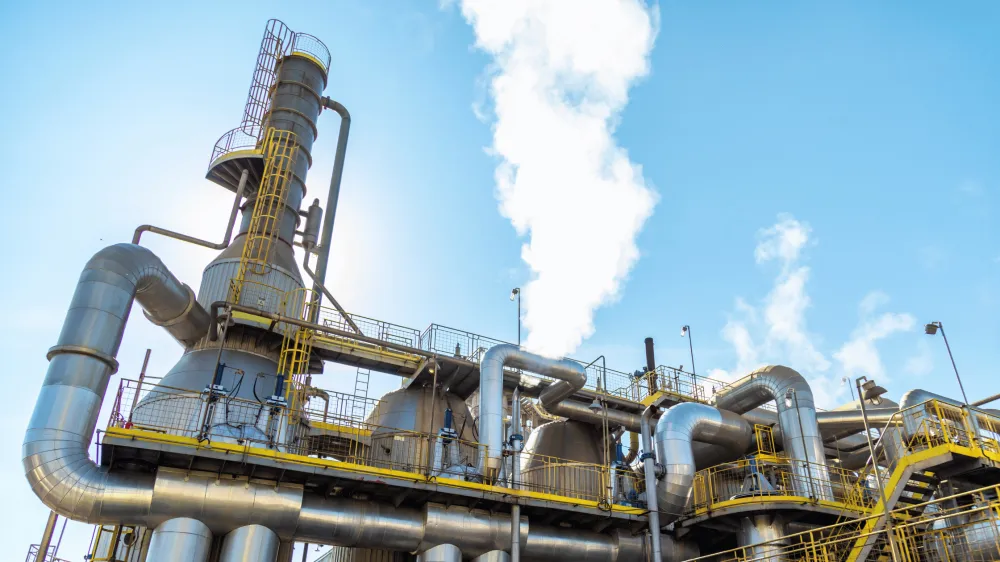# Constant Returns to Scale

Constant returns to scale refer to the phenomenon whereby the output of a production process increases in proportion to the inputs, regardless of the scale of the operation. In other words, if the inputs to a production process are doubled, the output will also double, regardless of the size of the operation.

Here the word ‘scale’ means the amount of all the factors of production (FOP) held by a firm including units of labor, land and capital. If the scale is doubled, it means all the factors of production are doubled. Firms can increase the scale only in the long run. Firms can increase the scale only in the long run. In the long run all the inputs are variables.

One way to understand constant returns to scale is to consider the long run production function, which describes the relationship between inputs and output in a production process and can be explained with the help of the law of returns to scale.

## The Law of Returns to Scale

If all the factors of production are increased with the same proportion i.e. the scale is increased in long run, then increase in the level of outputs can be of the following types.

### Increasing Returns to Scale

If an increase in all the factors of production by the same proportion (an increase in the scale) leads to more than proportionate increase in the output, then the change in the output is called increasing returns to scale. This means that the output is increased by a greater proportion than the increase in total input. It means the rate of change of output is more than the rate of change of inputs. This is the first category (variation) of returns to scale.

In above table, increasing returns to scale (IRS) are shown.

When all the FOP are increased from 1 to 2 then

% increase in FOP = [(2–1)/1] X 100% = 100% and

% increase in output Q (Shown on diagonal of table) = [(50–20)/20] X 100% = 150%.

When all the FOP are increased from 2 to 3 then

% increase in FOP = [(3–2)/2] X 100% = 50% and

% increase in output Q (Shown on diagonal of table) = [(80–50)/50] X 100% = 60%.

Again % increase in output is greater than % increase in the scale. Hence this is called increasing returns to scale.

### Constant Returns to Scale

If an increase in all the factors of production by the same proportion (an increase in the scale) leads to the same proportionate increase in the output, then the change in the output is called constant returns to scale. It means the rate of change of output is equal to the rate of change of inputs. This is the second category(variation) of returns to scale.

In above table, constant returns to scale (CRS) are shown.

When all the FOP are increased from 1 to 2 then

% increase in FOP = [(2–1)/1] X 100% = 100% and

% increase in the output Q (Shown on diagonal of table) = [(40–20)/20] X 100% = 100%

When all the FOP are increased from 2 to 3 then

% increase in FOP = [(3–2)/2] X 100% = 50% and

% increase in the output Q (Shown on diagonal of table)= [(60–40)/40] X 100% = 50%

Again % increase the output is equal to % increase in the scale. Hence this is constant returns to scale.

### Decreasing Returns to Scale

If an increase in all the factors of production by the same proportion (an increase in the scale) leads to less than proportionate increase in the output, then the change in the output is called decreasing returns of scale. It means the rate of change of output is less tha the rate of change of inputs. This is the third category(variation) of returns to scale.

In above table, decreasing returns to scale (DRS) are shown.

When all the FOP are increased from 1 to 2 then

% increase in FOP = [(2–1)/1] X 100% = 100% and

% increase in the output Q (Shown on diagonal of table) = [(30–20)/20] X 100% = 50%

When all the FOP are increased from 2 to 3 then

% increase in FOP = [(3–2)/2] X 100% = 50% and

% increase in the output Q (Shown on diagonal of table) = [(36–30)/30] X 100% = 20%

So % increase in the output is less than % increase in the scale. Hence this is decreasing returns to scale.

## Factors affecting the Returns to Scale

The level of technology and the efficiency of the production process can affect the returns to scale. If a company is able to adopt new technologies or improve the efficiency of its production process, it may experience increasing returns to scale, where the output increases at a faster rate as the inputs are increased. On the other hand, if a company is using out-dated technologies or has an inefficient production process, it may experience decreasing returns to scale, where the output increases at a slower rate as the inputs are increased.

The level of specialisation and division of labour within the organisation can affect the returns to scale. If a company is able to specialise and divide tasks among workers, it may be able to achieve increasing returns to scale, as the increased specialisation can lead to increased efficiency and productivity. On the other hand, if a company lacks specialisation and division of labour, it may experience decreasing returns to scale, as the lack of specialisation can lead to reduced efficiency and productivity.

## Returns to Scale and the Long Run Average Cost (LRAC)

One important aspect of constant returns to scale is its impact on the cost structure of a business. The diagram below shows long run average cost (LRAC). The quantity is taken on the X-axis and cost is taken on the Y-axis.